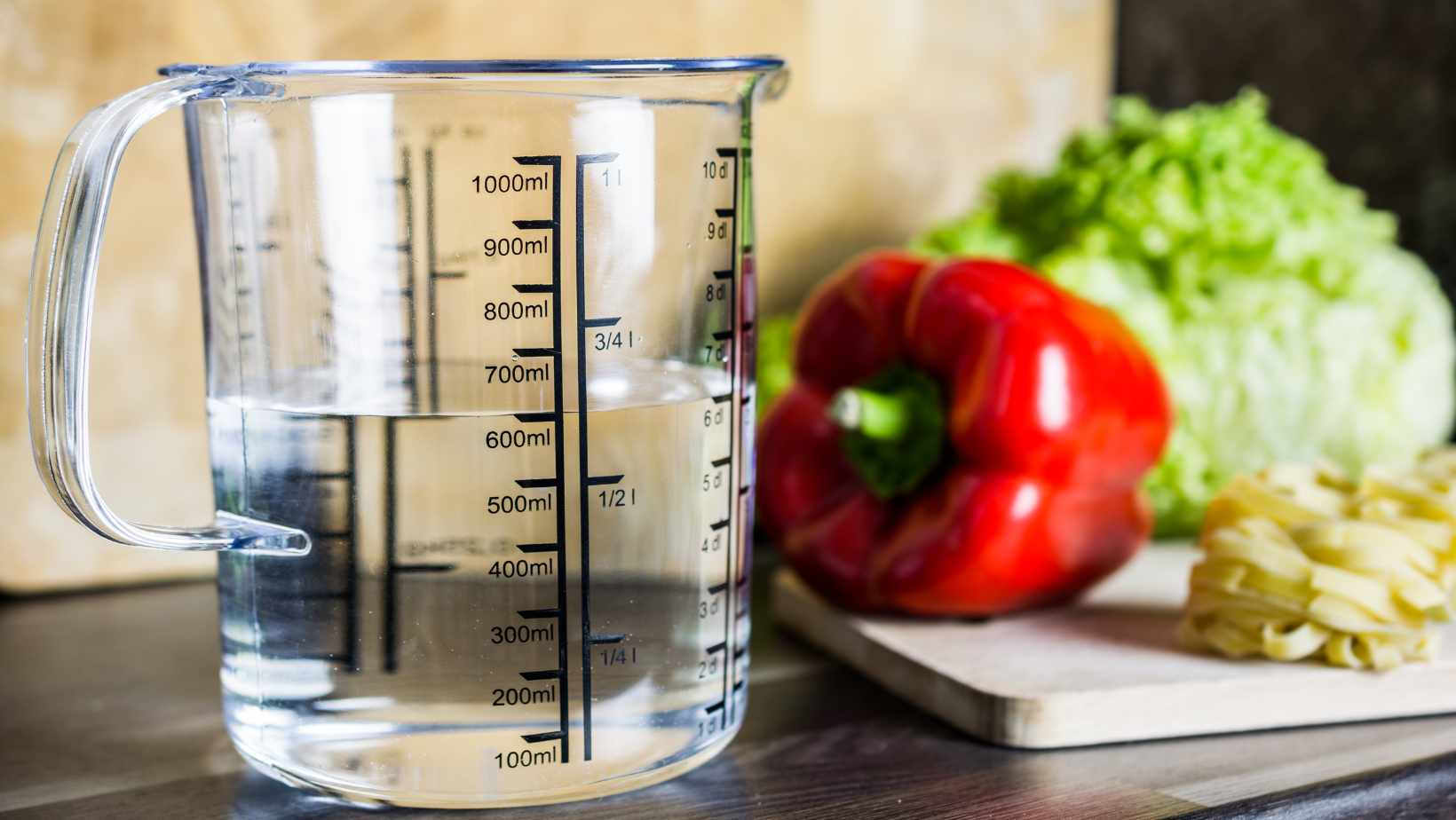# Find Out The Conversion: How Many ML in a CLIf you’ve ever found yourself wondering about the conversion between milliliters (ml) and centiliters (cl), you’re not alone. Understanding how many ml are in a cl can be quite useful, especially when it comes to precise measurements in cooking, bartending, or scientific experiments. Let’s dive right into it and clarify this common query.

To put it simply, there are 10 milliliters in a centiliter. Both milliliters and centiliters are units of volume measurement within the metric system, with “milli-” representing one thousandth and “centi-” representing one hundredth. Therefore, when converting from cl to ml, we multiply the number of centiliters by 10 to obtain the equivalent amount in milliliters.

## How Many ML In A CL

When it comes to measurements, the metric system is widely used across the globe for its simplicity and consistency. Unlike other measurement systems, such as the Imperial system, which can be a bit perplexing with its various units and conversions, the metric system offers a straightforward approach to measuring quantities. It’s based on multiples of ten, making it easy to convert between different units.

### Understanding Centiliters (cl)

Moving up from milliliters, we come across centiliters (cl). A centiliter is equal to one-hundredth of a liter or ten milliliters. While not as commonly used as liters or milliliters in everyday conversations, this unit still finds its place in certain contexts where larger volumes need more precision than whole liters can provide.

Some examples where centiliters might be utilized include:

• Measuring smaller amounts of liquid medicine.
• Estimating water consumption for plants that require specific watering amounts.
• Determining serving sizes for beverages in some countries where cl may be preferred over ml.

In summary, understanding the metric system lays the foundation for comprehending the relationship between different units of measurement. The milliliter (ml) serves as a fundamental unit for smaller volumes, while the centiliter (cl) provides more precision for larger quantities than liters alone. By grasping these concepts, you’ll be better equipped to navigate fluid measurements and conversions in various domains of life, whether it’s cooking, medicine, or simply understanding the labels on your favorite beverages.

Now that we’ve covered the basics of the metric system and explored milliliters (ml) and centiliters (cl), let’s dive deeper into other aspects related to these units and their conversions in the next sections. Stay tuned!

## What is a Milliliter?

A milliliter (ml) is a unit of measurement commonly used to quantify liquid volumes. It is a metric unit, and it’s derived from the liter, which is the base unit for volume in the International System of Units (SI). The prefix “milli-” signifies that a milliliter is equal to one-thousandth of a liter.

To put it simply, 1 milliliter is equivalent to 0.01 centiliters (cl) or 0.001 deciliters (dl). This means that there are 10 milliliters in a centiliter and 100 milliliters in a deciliter.

The convenience of using milliliters as a measure lies in its suitability for smaller quantities, especially when dealing with liquids such as medication doses or cooking ingredients. It allows for precise and accurate measurements without the need for excessive decimals or fractions.

In practical terms, you can think of a milliliter as being roughly equivalent to the volume contained within an average-sized medicine dropper or about 20 drops of water.

## Conversions

Understanding how many milliliters are in other common units can be helpful when working with different measuring systems:

• 1 milliliter (ml) = 0.001 liters (l): This conversion enables easy conversion between larger volumes like liters and smaller ones like milliliters.
• 1 centiliter (cl) = 10 milliliters: Centiliters are often used when measuring small amounts of liquid.
• 1 deciliter (dl) = 100 milliliters: Deciliters are useful when dealing with slightly larger quantities than centiliters but still smaller than liters.

Remember that converting between units involves multiplying or dividing by powers of ten, depending on whether you’re going from larger to smaller units or vice versa.

Here’s a quick reference table to summarize the conversions:

 Milliliters (ml) Centiliters (cl) Deciliters (dl) 1 ml 0.1 cl 0.01 dl 10 ml 1 cl 0.1 dl 100 ml 10 cl 1 dl

Understanding the relationship between milliliters, centiliters, and deciliters can be beneficial in various situations where precise measurements are required, such as cooking or scientific experiments.

In conclusion, a milliliter is a unit of measurement commonly used to quantify liquid volumes. It is equal to one-thousandth of a liter and offers an accurate way to measure smaller quantities. By understanding its conversion factors with other units like centiliters and deciliters, you can easily navigate different measuring systems and ensure precise measurements in your daily activities.

Amanda is the proud owner and head cook of her very own restaurant. She loves nothing more than experimenting with new recipes in the kitchen, and her food is always a big hit with customers. Amanda takes great pride in her work, and she always puts her heart into everything she does. She's a hard-working woman who has made it on her own, and she's an inspiration to all who know her.